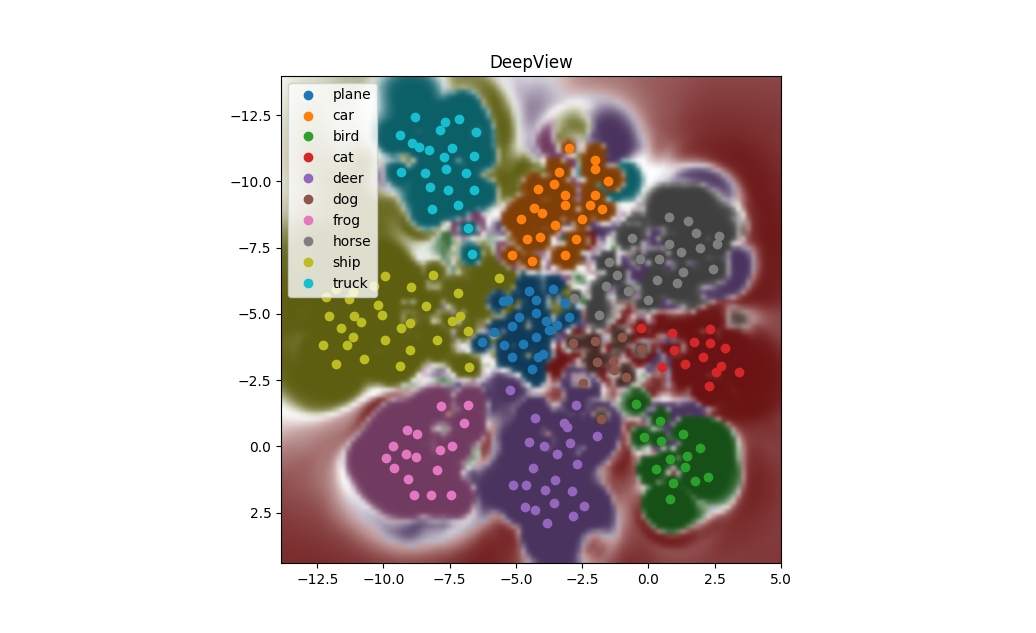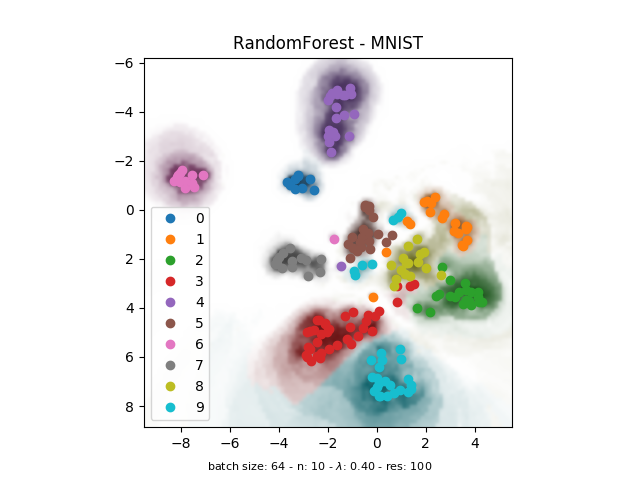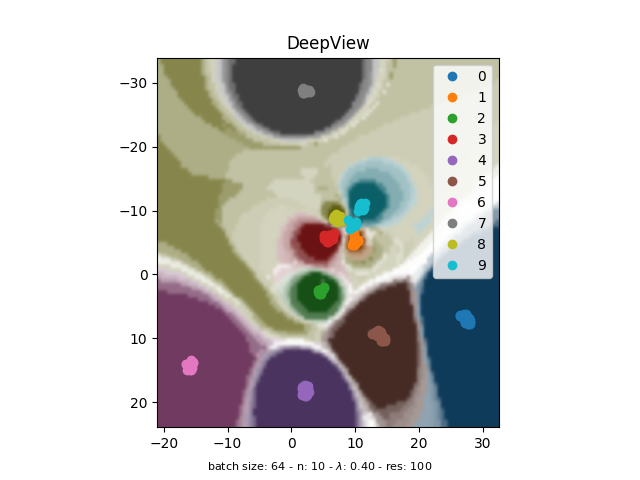DeepView Demo

# DeepView: Visualization of classifiers trained on high-dimensional data¶

Schulz A, Hinder F, Hammer B (2020)

Paper in the Proceedings of the Twenty-Ninth International Joint Conference on Artificial Intelligence: https://www.ijcai.org/Proceedings/2020/319, DOI: 10.24963/ijcai.2020/319

Get instruction to download the code from the ITS.ML GitHub, or access it directly at the DeepView GitHub!

In :
from deepview import DeepView
import matplotlib.pyplot as plt
import numpy as np
import time
# ---------------------------
import demo_utils as demo

%matplotlib qt

In :
# matplotlib qt seems to be a bit buggy with notebooks, so we execute it multiple times
%matplotlib qt


## Getting data and models¶

Each section in this notebook can be run independently, thus at the beginning of each section, the according model (i.e. torch/knn/decision tree) and the dataset will be initialized. The reason for this is, that running both torch and tensorflow simultaneously on the GPU may lead to problems. This notebook tests the DeepView framework on different classifiers

• ResNet-20 on CIFAR10
• DecisionTree on MNIST
• RandomForest on MNIST
• KNN on MNIST

## DeepView Usage Instructions¶

1. Create a wrapper funktion like pred_wrapper which receives a numpy array of samples and returns according class probabilities from the classifier as numpy arrays
2. Initialize DeepView-object and pass the created method to the constructor
3. Run your code and call add_samples(samples, labels) at any time to add samples to the visualization together with the ground truth labels.
• The ground truth labels will be visualized along with the predicted labels
• The object will keep track of a maximum number of samples specified by max_samples and it will throw away the oldest samples first
4. Call the show method to render the plot

The following parameters must be specified on initialization:

Variable

Meaning

(!)pred_wrapper

Wrapper function allowing DeepView to use your model. Expects a single argument, which should be a batch of samples to classify. Returns (valid / softmaxed) prediction probabilities for this batch of samples.

(!)classes

Names of all different classes in the data.

(!)max_samples

The maximum amount of samples that DeepView will keep track of. When more samples are added, the oldest samples are removed from DeepView.

(!)batch_size

The batch size used for classification

(!)data_shape

Shape of the input data (complete shape; excluding the batch dimension)

resolution

x- and y- Resolution of the decision boundary plot. A high resolution will compute significantly longer than a lower resolution, as every point must be classified, default 100.

cmap

Name of the colormap that should be used in the plots, default 'tab10'.

interactive

When interactive is True, this method is non-blocking to allow plot updates. When interactive is False, this method is blocking to prevent termination of python scripts, default True.

title

Title of the deepview-plot.

data_viz

DeepView has a reactive plot, that responds to mouse clicks and shows the according data sample, when it is clicked. You can pass a custom visualization function, if data_viz is None, DeepView will try to show each sample as an image, if possible. (optional, default None)

mapper

An object that maps samples from the data space to 2D space. Normally UMAP is used for this, but you can pass a custom mapper as well. (optional)

inv_mapper

An object that maps samples from the 2D space to the data space. Normally deepview.embeddings.InvMapper is used for this, but you can pass a custom inverse mapper as well. (optional)

kwargs

Configuration for the embeddings in case they are not specifically given in mapper and inv_mapper. Defaults to deepview.config.py. (optional)

## Demo with Torch model¶

In :
import torch

# device will be detected automatically
# Set to 'cpu' or 'cuda:0' to set the device manually
device = torch.device('cuda:0' if torch.cuda.is_available() else 'cpu')
# get torch model
torch_model = demo.create_torch_model(device)
# get CIFAR-10 data
testset = demo.make_cifar_dataset()

print('\nUsing device:', device)

Created PyTorch model:	 ResNet
* Dataset:		 CIFAR10
* Best Test prec:	 91.78000183105469

100.0%
Extracting data/cifar-10-python.tar.gz to data

Using device: cpu

In :
# softmax operation to use in pred_wrapper
softmax = torch.nn.Softmax(dim=-1)

# this is the prediction wrapper, it encapsulates the call to the model
# and does all the casting to the appropriate datatypes
def pred_wrapper(x):
x = np.array(x, dtype=np.float32)
tensor = torch.from_numpy(x).to(device)
logits = torch_model(tensor)
probabilities = softmax(logits).cpu().numpy()
return probabilities

def visualization(image, point2d, pred, label=None, title=None):
f, a = plt.subplots()
a.set_title(title)
a.imshow(image.transpose([1, 2, 0]))

# the classes in the dataset to be used as labels in the plots
classes = ('plane', 'car', 'bird', 'cat', 'deer', 'dog', 'frog', 'horse', 'ship', 'truck')

# --- Deep View Parameters ----
batch_size = 512
max_samples = 500
data_shape = (3, 32, 32)
n = 5
lam = .65
resolution = 100
cmap = 'tab10'
title = 'ResNet-20 - CIFAR10'

deepview = DeepView(pred_wrapper, classes, max_samples, batch_size,
data_shape, n, lam, resolution, cmap, title=title, data_viz=None)

In :
n_samples = 150
sample_ids = np.random.choice(len(testset), n_samples)
X = np.array([ testset[i].numpy() for i in sample_ids ])
Y = np.array([ testset[i] for i in sample_ids ])

t0 = time.time()
deepview.show()

print('Time to calculate visualization for %d samples: %.2f sec' % (n_samples, time.time() - t0))

Distance calculation 20.00 %
Distance calculation 40.00 %
Distance calculation 60.00 %
Distance calculation 80.00 %
Distance calculation 100.00 %
Embedding samples ...
Computing decision regions ...
Time to calculate visualization for 150 samples: 616.33 sec


## Add new samples to the visualization¶

In :
n_new = 200

sample_ids = np.random.choice(len(testset), n_new)
X = np.array([ testset[i].numpy() for i in sample_ids ])
Y = np.array([ testset[i] for i in sample_ids ])

t0 = time.time()
deepview.show()

print('Time to add %d samples to visualization: %.2f sec' % (n_new, time.time() - t0))

Distance calculation 20.00 %
Distance calculation 40.00 %
Distance calculation 60.00 %
Distance calculation 80.00 %
Distance calculation 100.00 %
Embedding samples ...
Computing decision regions ...
Time to add 200 samples to visualization: 7139.59 sec


### Example output¶

As the plot is updatable, it is shown in a separate Qt-window. With the CIFAR-data and the model loaded above, the following plot was produced after 200 samples where added:

Hyperparameters: n = 10 lam = 0.2 resolution = 100## Tuning the $\lambda$-Hyperparameter¶

The $\lambda$-Hyperparameter weights the euclidian distance component. When the visualization doesn't show class-clusters, try a smaller lambda to put more emphasis on the discriminative distance component that considers the class. A smaller $\lambda$ will pull the datapoints further into their class-clusters. Therefore, a too small $\lambda$ can lead to collapsed clusters that don't represent any structural properties of the datapoints. Of course this behaviour also depends on the data and how well the label corresponds to certain structural properties.

Due to separate handling of euclidian and class-discriminative distances, the $\lambda$ parameter can easily be adjusted. Distances don't need to be recomputed, only the embeddings and therefore also the plot of the decision boundary.

In :
deepview.set_lambda(.7)
deepview.show()

Embedding samples ...
Computing decision regions ...


## Compare performance¶

For this test, DeepView was run on a GPU (GTX 2060 6GB). Adding samples may be a bit more time consuming, then just running DeepView on the desired amount of samples to be visualized. This is because the decision boundaries must be calculated twice with a similar time complexity. However, the step of adding 100 samples to 100 existing samples takes less time then computing it from scratch for 200 samples. This is because distances were already computed for half of the samples and can be reused.

Szenario

Time

From scratch for 100 samples

31.20 sec

66.89 sec

From scratch for 200 samples

71.16 sec

200 samples when adding 100 samples in two steps

98.19 sec
In :
deepview.reset()

n_samples = 200
sample_ids = np.random.choice(len(testset), n_samples)
X = np.array([ testset[i].numpy() for i in sample_ids ])
Y = np.array([ testset[i] for i in sample_ids ])

t0 = time.time()
deepview.show()

print('Time to calculate visualization for %d samples: %.2f sec' % (n_samples, time.time() - t0))

Distance calculation 20.00 %
Distance calculation 40.00 %
Distance calculation 60.00 %
Distance calculation 80.00 %
Distance calculation 100.00 %
Embedding samples ...
Computing decision regions ...
Time to calculate visualization for 200 samples: 5687.20 sec


# Evaluate¶

These evaluations can be run with an initialized instance of DeepView.

In :
from deepview.evaluate import evaluate_umap

print('Evaluation of DeepView: %s\n' % deepview.title)
evaluate_umap(deepview, X, Y)

Evaluation of DeepView: ResNet-20 - CIFAR10

orig labs, knn err: eucl / fish 0.135 / 0.15
orig labs, knn err in proj space: eucl / fish 0.925 / 0.135
classif labs, knn err: eucl / fish 0.095 / 0.115
classif labs, knn acc in proj space: eucl / fish 10.5 / 90.5


## Evaluate the Inverse Mapping¶

Evaluation of the inverse mapping (i.e. the mapping from 2D back into sample-space) is done by first, passing some training samples to DeepView. It will classify them with the given model, train the mappers (UMAP and inverse) on them, and embed them into 2D space. A fraction of the embedded samples will be used to train the inverse mapper from ground up. After reconstructing the same set of samples, they will be classified again. The predictions are compared against the prior predictions from deepview and used to calculate the train accuracy.

The spare samples are used as testing samples, they were not used during training of the inverse mapper. They are mapped back into sample-space as well, classified and these classification are used to calculate the test accuracy of the inverse mapper.

To run this cell, run Demo with Torch model first, as the evaluation is done on the CIFAR dataset

In :
from deepview.evaluate import evaluate_inv_umap

# for testing, reset deepview and add some samples
# a fraction of these will serve as training set for the evaluation
n_samples = 600
fraction = 0.7

sample_ids = np.random.choice(len(testset), n_samples)
X = np.array([ testset[i].numpy() for i in sample_ids ])
Y = np.array([ testset[i] for i in sample_ids ])

train_acc, test_acc = evaluate_inv_umap(deepview, X, Y, fraction)

print('Inverse-Mapper train accuracy:\t%.2f%%' % train_acc)
print('Inverse-Mapper test accuracy:\t%.2f%%' % test_acc)

Distance calculation 20.00 %
Distance calculation 40.00 %
Distance calculation 60.00 %
Distance calculation 80.00 %
Distance calculation 100.00 %
Embedding samples ...
Computing decision regions ...
Inverse-Mapper train accuracy:	94.52%
Inverse-Mapper test accuracy:	82.78%

In :
deepview.close()


## Demo with Tensorflow And visualizing intermediate embeddings¶

This demo shows the usage of DeepView for tensorflow models (it doesn't differ at all from the procedure with torch models). However, this demo also shows how to feed intermediate embeddings of the data to DeepView. To do so, we only need to encode the datapoints before feeding them to DeepView. We proceed as follows:

1. Create a model that provides access to intermediate embeddings (i.e. output of some hidden layer)
2. Train the model, the example here is a simple feed forward neural network that reaches roughly 93.5% training accuracy
3. Encode the datapoints with the first layer(s) of the neural network into an embedding
4. Instantiate DeepView
1. The prediction wrapper now needs to be model_head, because the data samples will already be embedded by the first part of the model.
2. Instead of the raw data samples, feed the embedded data as input to DeepView
In :
import tensorflow as tf

verbose = 1

# get MNIST dataset
digits_X, digits_y = demo.make_digit_dataset()

# create a tensorflow models,
# model_embd will encode images to an intermediate embedding
# model_head will predict classes from the intermediate embedding
# model is model_embd and model_head combined into one model for training

_ = model.fit(digits_X, digits_y, batch_size=8, epochs=5, verbose=verbose)

Epoch 1/5
225/225 [==============================] - 0s 2ms/step - loss: 1.9236 - accuracy: 0.5481
Epoch 2/5
225/225 [==============================] - 0s 2ms/step - loss: 0.7955 - accuracy: 0.8191
Epoch 3/5
225/225 [==============================] - 0s 2ms/step - loss: 0.4342 - accuracy: 0.8965
Epoch 4/5
225/225 [==============================] - 1s 2ms/step - loss: 0.3039 - accuracy: 0.9238
Epoch 5/5
225/225 [==============================] - 0s 2ms/step - loss: 0.2347 - accuracy: 0.9366

In :
# Get the embedded data
n_samples = 300
sample_ids = np.random.choice(len(digits_X), n_samples)

# Encode the digits with the first two layers
embedded_digits = model_embd.predict(digits_X, batch_size=64)

X = np.array([ embedded_digits[i] for i in sample_ids ])
Y = np.array([ digits_y[i] for i in sample_ids ])

In :
# note that here, the head (last layers) must be used in the prediction wrapper,
# as we want to pass the embedded data to deepview

# the digit dataset is used, so classes are [0..9]
classes = np.arange(10)

# --- Deep View Parameters ----
batch_size = 64
max_samples = 500
sample_shape = (64,)
n = 10
lam = 0.5
resolution = 100
cmap = 'tab10'
title = 'TF-Model - Embedded MNIST'

# create DeepView object
deepview = DeepView(pred_wrapper, classes, max_samples, batch_size, sample_shape,
n, lam, resolution, cmap, title=title, data_viz=demo.mnist_visualization)

t0 = time.time()
deepview.show()

print('Time to calculate visualization for %d samples: %.2f sec' % (n_samples, time.time() - t0))

Distance calculation 20.00 %
Distance calculation 40.00 %
Distance calculation 60.00 %
Distance calculation 80.00 %
Distance calculation 100.00 %
Embedding samples ...
Computing decision regions ...
Time to calculate visualization for 300 samples: 37.70 sec


### Just an embedding ...¶

To visualize the embedding purely based on the euclidian distances between the embedded vectors, you can use $\lambda = 1$. In this case DeepView will ignore the fisher distance from the probabilities and produce just a 2D representation of the embedded vectors. This corresponds to applying UMAP on the data-embedding.

In :
deepview.set_lambda(1.)
deepview.show()

Embedding samples ...
Computing decision regions ...

In :
deepview.close()


## Demo with RandomForest¶

In :
# get MNIST dataset
digits_X, digits_y = demo.make_digit_dataset()
# initialize random forest
random_forest = demo.create_random_forest(digits_X, digits_y, n_estimators=100)

Created random forest
* No. of Estimators:	 100
* Dataset:		 MNIST
* Train score:		 1.0

In :
pred_wrapper = DeepView.create_simple_wrapper(random_forest.predict_proba)

# the digit dataset is used, so classes are [0..9]
classes = np.arange(10)

# --- Deep View Parameters ----
batch_size = 64
max_samples = 500
sample_shape = (64,)
n = 10
lam = 0.5
resolution = 100
cmap = 'tab10'
title = 'RandomForest - MNIST'

# create DeepView object
deepview = DeepView(pred_wrapper, classes, max_samples, batch_size, sample_shape,
n, lam, resolution, cmap, title=title, data_viz=demo.mnist_visualization)

n_samples = 50
sample_ids = np.random.choice(len(digits_X), n_samples)
X = np.array([ digits_X[i] for i in sample_ids ])
Y = np.array([ digits_y[i] for i in sample_ids ])

t0 = time.time()
deepview.show()

print('Time to calculate visualization for %d samples: %.2f sec' % (n_samples, time.time() - t0))

Distance calculation 20.00 %
Distance calculation 40.00 %
Distance calculation 60.00 %
Distance calculation 80.00 %
Distance calculation 100.00 %
Embedding samples ...
Computing decision regions ...
Time to calculate visualization for 50 samples: 12.01 secIn :
deepview.close()


## Demo with DecisionTree¶

In :
# get MNIST dataset
digits_X, digits_y = demo.make_digit_dataset()
# initialize decision tree
decision_tree = demo.create_decision_tree(digits_X, digits_y, max_depth=10)

Created decision tree
* Depth:		 10
* Dataset:		 MNIST
* Train score:		 0.9833055091819699

In :
# --- Deep View Parameters ----
batch_size = 256
max_samples = 500
# the data can also be represented as a vector
sample_shape = (64,)
n = 10
lam = 0.65
resolution = 100
cmap = 'gist_ncar'

# the digit dataset is used, so classes are [0..9]
classes = np.arange(10)

In :
pred_wrapper = DeepView.create_simple_wrapper(decision_tree.predict_proba)

# create DeepView object
deepview = DeepView(pred_wrapper, classes, max_samples, batch_size, sample_shape,
n, lam, resolution, cmap, data_viz=demo.mnist_visualization)

n_samples = 200
sample_ids = np.random.choice(len(digits_X), n_samples)
X = np.array([ digits_X[i] for i in sample_ids ])
Y = np.array([ digits_y[i] for i in sample_ids ])

t0 = time.time()
deepview.show()

print('Time to calculate visualization for %d samples: %.2f sec' % (n_samples, time.time() - t0))

Distance calculation 20.00 %
Distance calculation 40.00 %
Distance calculation 60.00 %
Distance calculation 80.00 %
Distance calculation 100.00 %
Embedding samples ...
Computing decision regions ...
Time to calculate visualization for 200 samples: 16.67 sec

In :
deepview.set_lambda(.4)
deepview.show()

Embedding samples ...
Computing decision regions ...

In :
deepview.close()


## Demo: KNN-Classifier¶

In :
# get MNIST dataset
digits_X, digits_y = demo.make_digit_dataset()
# initialize knn classifier
kn_neighbors = demo.create_kn_neighbors(digits_X, digits_y, k=10)

Created knn classifier
* No. of Neighbors:	 10
* Dataset:		 MNIST
* Train score:		 0.9855314412910406

In :
pred_wrapper = DeepView.create_simple_wrapper(kn_neighbors.predict_proba)

# create DeepView object
deepview = DeepView(pred_wrapper, classes, max_samples, batch_size, sample_shape,
n, lam, resolution, cmap, data_viz=demo.mnist_visualization)

n_samples = 200
sample_ids = np.random.choice(len(digits_X), n_samples)
X = np.array([ digits_X[i] for i in sample_ids ])
Y = np.array([ digits_y[i] for i in sample_ids ])

t0 = time.time()
deepview.show()

print('Time to calculate visualization for %d samples: %.2f sec' % (n_samples, time.time() - t0))

Distance calculation 20.00 %
Distance calculation 40.00 %
Distance calculation 60.00 %
Distance calculation 80.00 %
Distance calculation 100.00 %
Embedding samples ...
Computing decision regions ...
Time to calculate visualization for 200 samples: 94.62 secIn :
deepview.close()